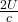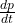## We know the mass and luminosity of the star. We also know the mass of the spacecraft and the area of its stellar sail. We must find the net

Question

We know the mass and luminosity of the star. We also know the mass of the spacecraft and the area of its stellar sail. We must find the net force exerted on the spacecraft, as well as the direction of its acceleration. We are asked to find both the net force exerted on the spacecraft and the direction of the acceleration. Which principle is best used to find the direction of the acceleration once the net force has been found

in progress 0
6 months 2021-08-15T13:20:16+00:00 1 Answers 6 views 0

## Answers ( )

Newton’s principle of force, the variation of the momentum and the acceleration have the same direction

Explanation:

To find the force exerted by the star on the sail of the ship, the expression of the momentum of the radiation is used, which in the case of a total reflection

p =where p is the momentum and U the incident energy and c the speed of light.

The strength I could get from

F =the direction of the acceleration is the direction of the momentum, therefore the principle to find the acceleration of is Newton’s principle of force, the variation of the momentum and the acceleration have the same direction.